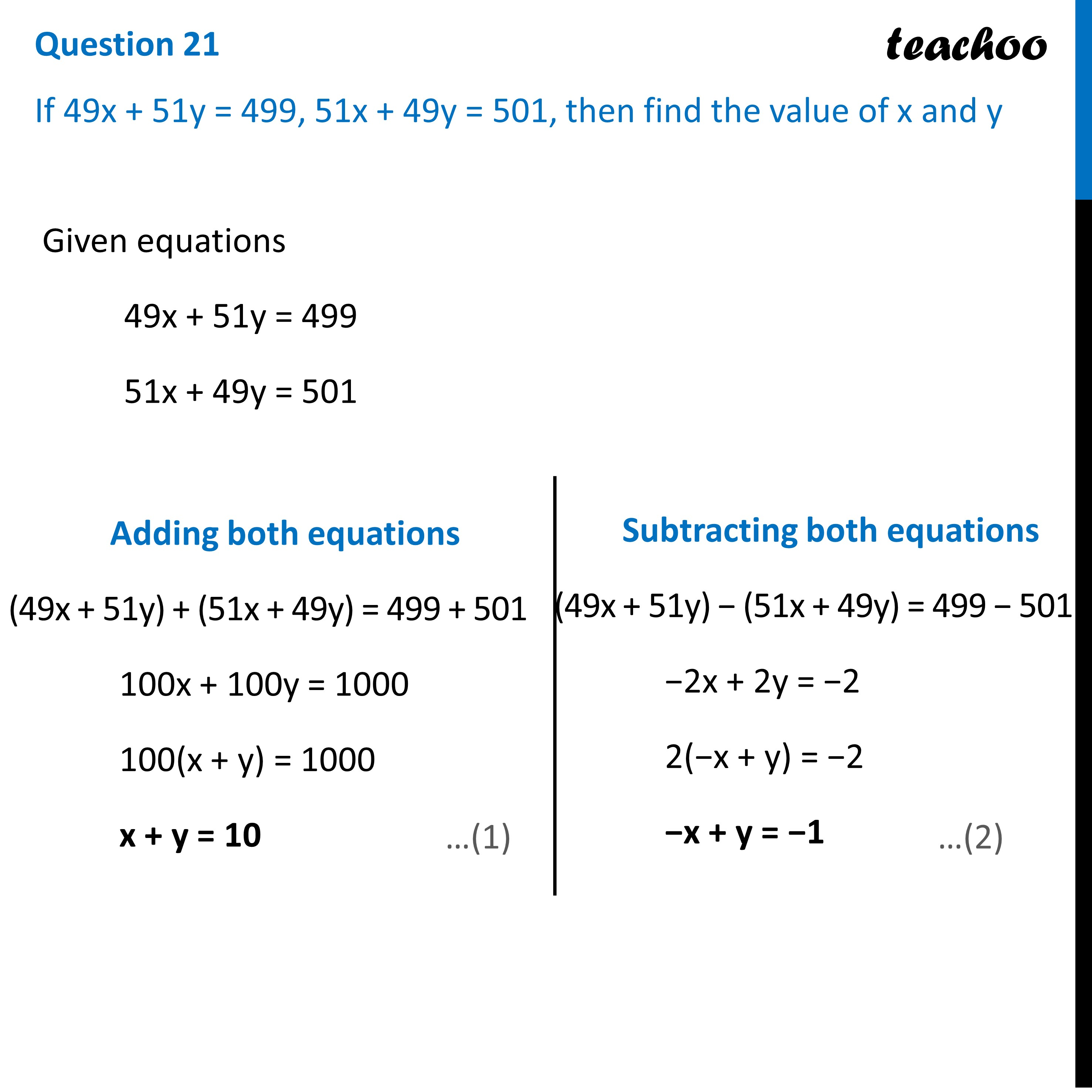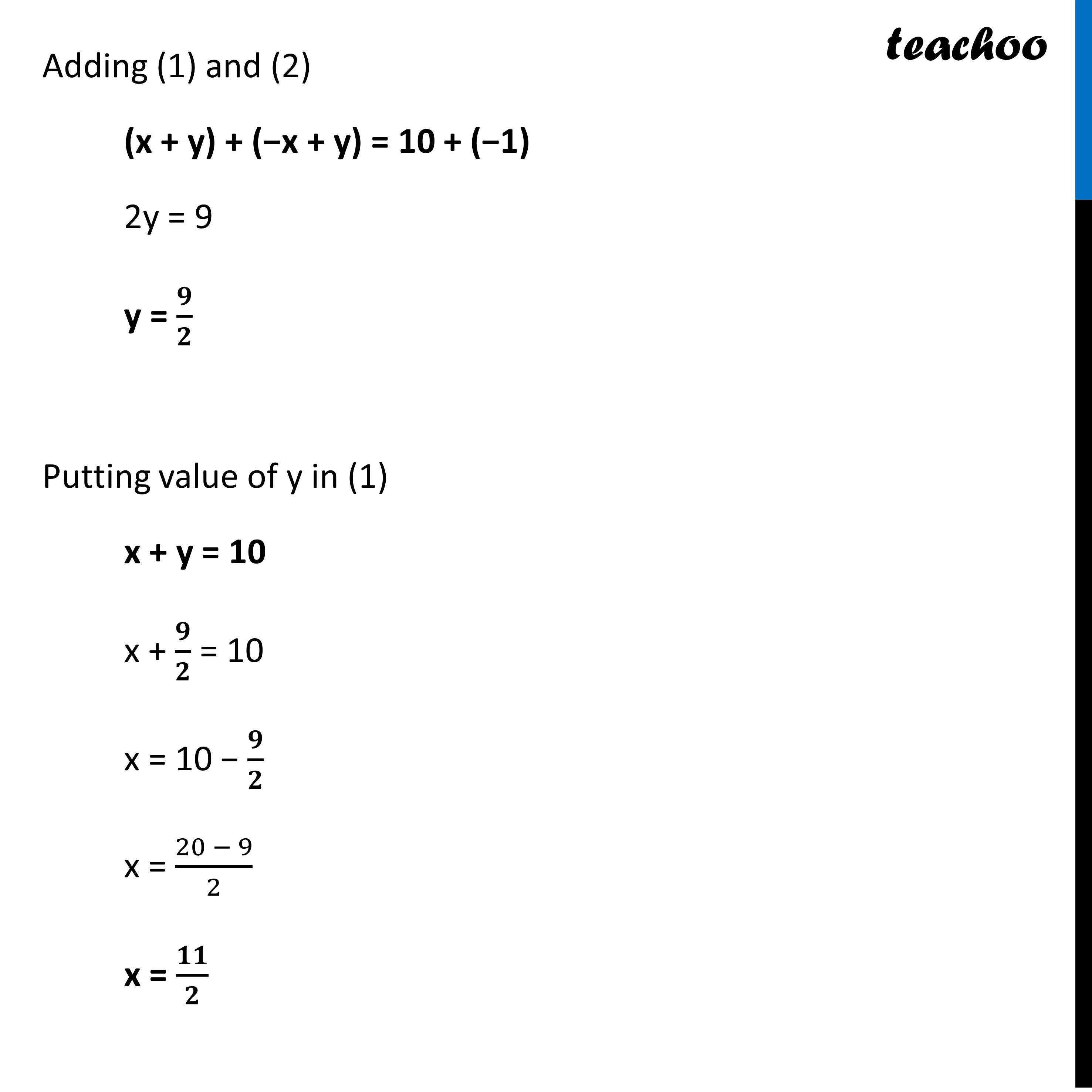CBSE Class 10 Sample Paper for 2023 Boards - Maths Standard

Class 10
Solutions of Sample Papers for Class 10 Boards

## If 49x + 51y = 499, 51x + 49y = 501, then find the value of x and y.Learn in your speed, with individual attention - Teachoo Maths 1-on-1 Class

### Transcript

Question 21 If 49x + 51y = 499, 51x + 49y = 501, then find the value of x and yGiven equations 49x + 51y = 499 51x + 49y = 501 Adding both equations (49x + 51y) + (51x + 49y) = 499 + 501 100x + 100y = 1000 100(x + y) = 1000 x + y = 10 Subtracting both equations (49x + 51y) − (51x + 49y) = 499 − 501 −2x + 2y = −2 2(−x + y) = −2 −x + y = −1 Adding (1) and (2) (x + y) + (−x + y) = 10 + (−1) 2y = 9 y = 𝟗/𝟐 Putting value of y in (1) x + y = 10 x + 𝟗/𝟐 = 10 x = 10 − 𝟗/𝟐 x = (20 − 9)/2 x = 𝟏𝟏/𝟐